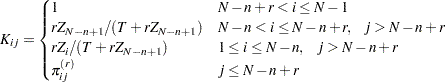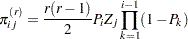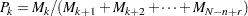### PPS Sampling without Replacement

If you specify the option METHOD=PPS, PROC SURVEYSELECT selects units with probability proportional to size and without replacement. The selection probability for unitin stratumequals, where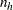is the sample size for stratum, and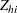is the relative size of unitin stratum. The relative size equals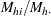, which is the ratio of the size measure for unitin stratum(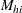) to the total of all size measures for stratum(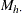).

Because selection probabilities cannot exceed 1, the relative size for each unit must not exceed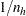for METHOD=PPS. This requirement can be expressed as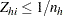, or equivalently,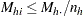. If your size measures do not meet this requirement, you can adjust the size measures by using the MAXSIZE= or MINSIZE= option. Or you can request certainty selection for the larger units by using the CERTSIZE= or CERTSIZE=P= option. Alternatively, you can use a selection method that does not have this relative size restriction, such as PPS with minimum replacement (METHOD=PPS_SEQ).

PROC SURVEYSELECT uses the Hanurav-Vijayan algorithm for PPS selection without replacement. Hanurav (1967) introduced this algorithm for the selection of two units per stratum, and Vijayan (1968) generalized it for the selection of more than two units. The algorithm enables computation of joint selection probabilities and provides joint selection probability values that usually ensure nonnegativity and stability of the Sen-Yates-Grundy variance estimator. See Fox (1989), Golmant (1990), and Watts (1991) for details.

Notation in the remainder of this section drops the stratum subscriptfor simplicity, but selection is still done independently within strata if you specify a stratified design. For a stratified design,now denotes the sample size for the current stratum,
denotes the stratum population size, and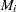denotes the size measure for unitin the stratum. If the design is not stratified, this notation applies to the entire sampling frame.

According to the Hanurav-Vijayan algorithm, PROC SURVEYSELECT first orders units within the stratum in ascending order by size measure, so that. Then the procedure selects the PPS sample ofobservations as follows:

1. The procedure randomly chooses one of the integers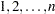with probability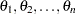, where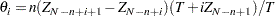where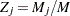and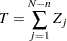By definition,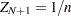to ensure that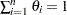.

2. Ifis the integer selected in step 1, the procedure includes the last (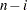) units of the stratum in the sample, where the units are ordered by size measure as described previously. The procedure then selects the remainingunits according to steps 3 through 6.

3. The procedure defines new normed size measures for the remaining (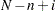) stratum units that were not selected in steps 1 and 2: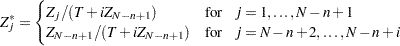4. The procedure selects the next unit from the first (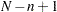) stratum units with probability proportional to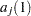, where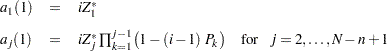and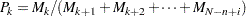5. If stratum unit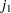is the unit selected in step 4, then the procedure selects the next unit from units (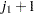) through (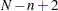) with probability proportional to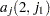, where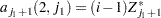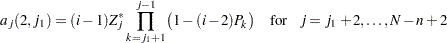6. The procedure repeats step 5 until allsample units are selected.

If you specify the JTPROBS option, PROC SURVEYSELECT computes the joint selection probabilities for all pairs of selected units in each stratum. The joint selection probability for unitsandin the stratum equals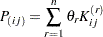where